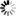Normal view

## Nonparametric statistics with applications to science and engineering / Paul H. Kvam, Brani Vidakovic

Material type:TextPublication details: Hoboken, N.J Wiley-Interscience cop. 2007Description: xiv, 420 s. ill., diagr., tab. 25 cmISBN: 9780470081471 (inb)DDC classification: 519.5 Other classification: Thi | 62P30 | 62H17 | 62-01 | 62G | 519.5 | 62
Contents:
Introduction -- Probability basics -- Statistics basics -- Bayesian statistics -- Order statistics -- Goodness of fit -- Rank tests -- Designed experiments -- Categorical data -- Estimating distribution functions -- Density estimation -- Beyond linear regression -- Curve fitting techniques -- Wavelets -- Bootstrap -- Em algorithm -- Statistical learning -- Nonparametric bayes
Tags from this library: No tags from this library for this title.Average rating: 0.0 (0 votes)
Item type Current library Call number Status Date due Barcode
Book (loan)
519.5 (Browse shelf (Opens below)) Available 85001942689

Introduction -- Probability basics -- Statistics basics -- Bayesian statistics -- Order statistics -- Goodness of fit -- Rank tests -- Designed experiments -- Categorical data -- Estimating distribution functions -- Density estimation -- Beyond linear regression -- Curve fitting techniques -- Wavelets -- Bootstrap -- Em algorithm -- Statistical learning -- Nonparametric bayes

There are no comments on this title.